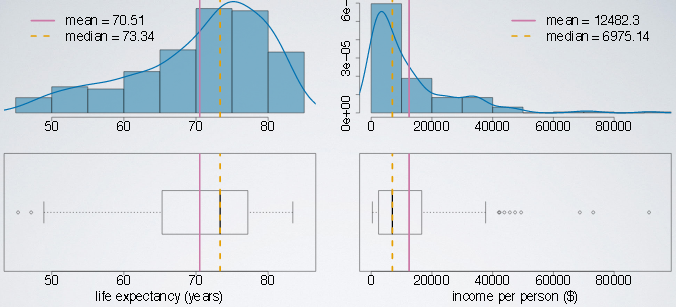### Center

• Mean: Arithmetic average;
• Sample mean is denoted as x bar;
• Population mean is denoted as Mu;
• Median: Midpoint of the distribution (50th percentile);
• Mode: Most frequent observation;

Sample statistics are point estimates of the population parameters. These estimates may not be perfect, but if the sample is good (representative of the population) they are usually good guesses.

#### Example 1: Odd number of observation• Mean: (75 + 69 +88 + 93 + 95 + 54 + 87 + 88 + 64)/9 = 75.11
• Mode: 88 (The most frequent observed value, in this case it is 88)
• Median: 87 (To do this, firstly sort the data in increasing order, then we find the mid-point of the ordered data)

#### Example 2: Even number of observationIn this case, we have 87 and 88 at the middle of our distribution.

• Median: (87 + 88)/2 = 87.5

### Skewness vs. Measures of Center

• In left skewed distributions, the lower valued observations pull the mean to themselves, and therefore the mean is generally lower than the median.
• In a symmetric distribution, the mean and the median are roughly equal to each other in the center of the distribution.
• In right skewed distributions, the high valued observations pull the mean, the arithmetic average to themselves, and therefore the mean is generally larger than the median.An example is shown below:• N/A